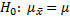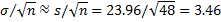# Hypothesis Testing using the Central Limit Theorem

Using the Central Limit Theorem we can extend the approach employed in Single Sample Hypothesis Testing for normally distributed populations to those that are not normally distributed. Suppose we take a sample of size n, where n is sufficiently large, and pose a null hypothesis that the population mean is the same as the sample mean; i.e.If we assume the null hypothesis, we know from the Central Limit Theorem that the sample mean has a normal distributionThis approach works well provided the variance of the population is known, which is not so common.

As we saw in Property 3 of Estimators, the variance of the sample is an unbiased estimator of the variance of the population, and so when the variance of the population is not known, we can estimate σ2 by s2.

As was done in Confidence Intervals for Sampling Distributions we can now set a confidence interval for the population mean as follows:

Excel Function: Excel provides the following two functions that can be useful in hypothesis testing.

ZTEST(R, μ0σ) = 1 – NORMDIST(, μ0$sigma/!sqrt{n}$, TRUE) where  = AVERAGE(R) = the sample mean of the data in range R and n = COUNT(R) = sample size. The third parameter is optional; when it is omitted the value of the sample standard deviation of R is used instead; i.e. ZTEST(R, μ0) = ZTEST(R, μ0s) where s = sample standard deviation = STDEV(R).

CONFIDENCE(ασn) = k such that ( – k + k) is the confidence interval for the mean based on the normal distribution; i.e. CONFIDENCE(ασn) = zcrit∙ std err, where n = sample size, σ = population standard deviation (or sample standard deviation s used as an estimate for σ) and 1 – α is the confidence %

Excel 2010/2013 also provide two additional functions Z.TEST and CONFIDENCE.NORM which are equivalent to ZTEST and CONFIDENCE respectively.

Observation: ZTEST(R, μ0σ) represents the probability that the true sample mean is greater than the observed sample mean AVERAGE(R) under the assumption that the population mean is μ0. This is a right tail test (i.e. it assumes that  ≥ μ0). If  < μ0 then ZTEST will return a value > .5.

If a left-tail test is desired (assuming  ≤ μ0), then use 1 – ZTEST(R, μ0σ). If a two-tail test is desired then use 2 * MIN(ZTEST(R, μ0σ), 1 – ZTEST(R, μ0σ)).

Observation: ZTEST and Z.TEST ignore any empty cells and cells with non-numeric values.

Observation: We could have calculated the confidence interval in Example 1 of Confidence Intervals for Sampling Distributions as follows:

CONFIDENCE(.05, 20, 60) = 5.06, and so the 95% confidence interval is (75 – 5.06, 75 + 5.06) = (69.94, 80.06).

Example 1: A company selling batteries claims that the average life for its batteries before a recharge is necessary is at least 100 hours. One of its clients wanted to verify this claim by testing 48 batteries as described in the Figure 1. Is the company’s claim correct?Figure 1 – One sample testing of the mean using ZTEST and CONFIDENCE

We test the following null hypothesis:

H0 ≤ 100

Since the sample size is sufficiently large (n = 48 ≥ 30), based on the Central Limit Theorem, the sampling distribution of the mean should be approximately normal with distribution N($sigma/!sqrt{n}$). Since the population standard deviation is not known we use the sample standard deviation (23.96) as an estimate for σ, and so the standard error isSince the sample mean  = 103.81, assuming the null hypothesis we can compute the p-value as follows:

p-value = 1 – NORMDIST(103.81, 100, 3.46, TRUE) = .135 > .05 = α

Since p-value > α, we cannot reject the null hypothesis, and so conclude there is not enough evidence to show that the company’s claim is false. Alternatively, we can arrive at the same result by using the ZTEST as follows:

p-value = ZTEST(A3:F10, 100) = .135 > .05 = α

Observation: If we had run a two-tail test, we could calculate CONFIDENCE(αsn) = CONFIDENCE(.05, 23.96, 48) = 6.78, and so the 95% confidence interval is (103.81 – 6.78, 103.81 + 6.78) = (97.03, 110.59). Since the hypothetical mean of 100 lies in this interval, we must retain the null hypothesis.

Real Statistics Excel Functions: The Real Statistics Resource Pack contains the following supplemental functions:

STDERR(R1) = STDEV(R1) / SQRT(COUNT(R1)), i.e. standard error for the data in range R1

NORM_CONF(R1, α) = CONFIDENCE(α, STDEV(R1), COUNT(R1))

NORM_LOWER(R1, α) = AVERAGE(R1) – NORM_CONF(R1, α)

NORM_UPPER(R1, α) = AVERAGE(R1) + NORM_CONF(R1, α)

Observation: All these functions ignore any empty cells and cells with non-numeric values. If α is omitted it defaults to .05.

Observation: For Example 1, we have STDERR(A3:F10) = 3.46, NORM_CONF(A3:F10, .05) = 6.78, NORM_LOWER(A3:F10, .05) = 97.03 and NORM_UPPER(A3:F10, .05) = 110.59.

Statistics for Beginners in Excel – AUC Confidence Interval

## Statistics for Beginners in Excel – Hypothesis Testing using the Central Limit Theorem

#### Free Machine Learning & Data Science Coding Tutorials in Python & R for Beginners. Subscribe @ Western Australian Center for Applied Machine Learning & Data Science.

Introduction to Applied Machine Learning & Data Science for Beginners, Business Analysts, Students, Researchers and Freelancers with Python & R Codes @ Western Australian Center for Applied Machine Learning & Data Science (WACAMLDS) !!!

Latest end-to-end Learn by Coding Projects (Jupyter Notebooks) in Python and R:

Applied Statistics with R for Beginners and Business Professionals

Data Science and Machine Learning Projects in Python: Tabular Data Analytics

Data Science and Machine Learning Projects in R: Tabular Data Analytics

Python Machine Learning & Data Science Recipes: Learn by Coding

R Machine Learning & Data Science Recipes: Learn by Coding

There are 2000+ End-to-End Python & R Notebooks are available to build Professional Portfolio as a Data Scientist and/or Machine Learning Specialist. All Notebooks are only \$79.95. We would like to request you to have a look at the website for FREE the end-to-end notebooks, and then decide whether you would like to purchase or not.

Please do not waste your valuable time by watching videos, rather use end-to-end (Python and R) recipes from Professional Data Scientists to practice coding, and land the most demandable jobs in the fields of Predictive analytics & AI (Machine Learning and Data Science).

The objective is to guide the developers & analysts to “Learn how to Code” for Applied AI using end-to-end coding solutions, and unlock the world of opportunities!

`Disclaimer: The information and code presented within this recipe/tutorial is only for educational and coaching purposes for beginners and developers. Anyone can practice and apply the recipe/tutorial presented here, but the reader is taking full responsibility for his/her actions. The author (content curator) of this recipe (code / program) has made every effort to ensure the accuracy of the information was correct at time of publication. The author (content curator) does not assume and hereby disclaims any liability to any party for any loss, damage, or disruption caused by errors or omissions, whether such errors or omissions result from accident, negligence, or any other cause. The information presented here could also be found in public knowledge domains.`